#CHANGING THE BASE OF A LOGARITHMLet a, b, and x be positive real numbers such that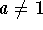and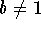(remember x must be greater than 0). Then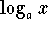can be converted to the base b by the formula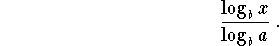Let's verify this with a few examples.

Example 1: Find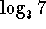to an accuracy of six decimals. Note that the answer will be between 1 and 2 because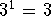and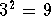, and 7 is between 3 and 9. According to the change of logarithm rule,can be written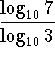.

When the base is 10, we can leave off the 10 in the notation. Thereforecan be written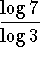. Using your calculator,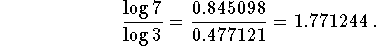You will note that the answer is between 1 and 2.

Let's check the answer. If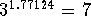, our answer is correct.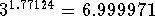. Close enough. Why isn't it 7 exactly? Well we roundedto six places, so our answer won't check exactly. If we rounded to ten places, then when we checked the answer, it would be closer to 7 than this answer.

Example 2: We could work the same problem by converting to the base e. According to the change of logarithm rule,can be written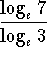. When the base is e, we can leave off the e in the notation andcan be written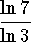. Using your calculator,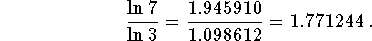You will note that the answer is between 1 and 2.

Example 3: Find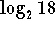. We know that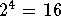and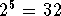, and that 18 is between 16 and 32; therefore, we know that the exponent we are looking for is between 4 and 5. In fact, it is closer to 4 than to 5 because 18 is closer to 16 than it is to 32.

Let's solve this problem by changing the base to 10.can be written. Using your calculator,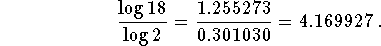Let's check the answer. If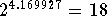, our answer is correct.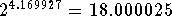. Close enough. Why isn't it 18 exactly? Since we roundedto six places, our answer won't check exactly. If we rounded to ten places, then when we checked the answer, it would be closer to 18 than this answer.

Example 4: We could work the problem in Example 3 by converting to the base e. According to the logarithmic rule,can be written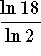. Using your calculator,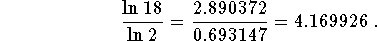The answer is the same as the answer you found when you converted the base to 10.

If you would like to review more examples of changing the base of a logarithm, click on Example.

Work the following problems and click on answer if you want to check your answer and review the solution.

Problem 1: Find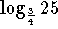.

Problem 2: Find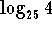.

Problem 3: Find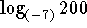.

Problem 4: Convert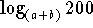to the base 2.[Algebra] [Trigonometry] [Complex Variables]S.O.S MATHematics home page

Do you need more help? Please post your question on our S.O.S. Mathematics CyberBoard.Author: Nancy Marcus